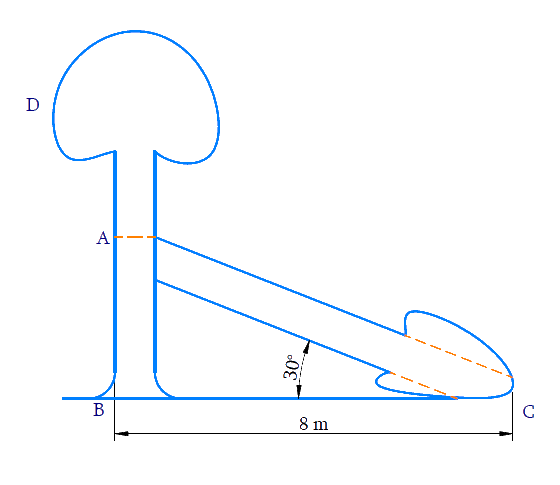# Ex.9.1 Q2 Some Applications of Trigonometry Solution - NCERT Maths Class 10

Go back to  'Ex.9.1'

## Question

A tree breaks due to storm and the broken part bends so that the top of the tree touches the ground making an angle $$30^{\circ}$$ with it. The distance between the foot of the tree to the point where the top touches the ground is $$8\,\rm{m}$$. Find the height of the tree.

Video Solution
Some Applications Of Trigonometry
Ex 9.1 | Question 2

## Text Solution

#### What is Known?

(i) Broken part of the tree bends and touching the ground making an angle of $$30^{\circ}$$ with the ground.

(ii) Distance between foot of the tree to the top of the tree is $$8\,\rm{m.}$$

#### What is Unknown?

Height of the tree#### Reasoning:

(i) Height of the tree $$= AB + AC.$$

(ii) Trigonometric ratio which involves $$AB, BC$$ and $$\angle C$$ is $$\tan \theta$$ , where $$AB$$ can be measured.

(iii) Trigonometric ratio which involves $$AB, AC$$ and $$\angle C$$ is $$\sin \theta$$ , where $$AC$$ can be measured.

(iv) Distance between the foot of the tree to the point where the top touches the ground $$= BC = 8\,\rm{ m}$$

#### Steps:

In $$\Delta ABC$$,

\begin{align}\tan C &=\frac{A B}{B C} \\ \tan 30^{\circ} &=\frac{A B}{8} \\ \frac{1}{\sqrt{3}} &=\frac{A B}{8} \\ A B &=\frac{8}{\sqrt{3}} \mathrm{m} \end{align}

\begin{align}\sin \,C{\rm{ }} &= {\rm{ }}\frac{{AB}}{{AC}}\\\sin 30^\circ &= \left( {\frac{{\frac{8}{{\sqrt 3 }}}}{{AC}}} \right)\\\frac{1}{2}{\rm{ }} &= {\rm{ }}\frac{8}{{\sqrt 3 }} \times \frac{1}{{AC}}\\AC{\rm{ }} &= {\rm{ }}\frac{8}{{\sqrt 3 }} \times 2\\AC{\rm{ }} &= {\rm{ }}\frac{{16}}{{\sqrt 3 }}\,\end{align}

\begin{align}\text{Height of tree}\, &= \rm{ }AB + AC\\&= \frac{8}{{\sqrt 3 }}+ \frac{{16}}{{\sqrt 3 }}\\&= \frac{{24}}{{\sqrt 3 }}\\&= \frac{{24}}{{\sqrt 3 }} \times \frac{{\sqrt 3 }}{{\sqrt 3 }}\\&= \frac{{24\sqrt 3 }}{3}\\&= 8\sqrt 3 \end{align}

Height of tree $$=8 \sqrt{3} \rm{m}$$

Learn from the best math teachers and top your exams

• Live one on one classroom and doubt clearing
• Practice worksheets in and after class for conceptual clarity
• Personalized curriculum to keep up with school